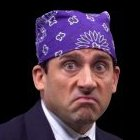Literature Quiz / Literature Without 'Literature'

Random Literature or Novel Quiz

Can you name these books that have had the letters L, I, T, E, R, A, T, U, R, and E removed?

byWalshyMusic  Plays Quiz Updated Feb 19, 2019

Score
0/20
Timer
04:00
W _ _ _ _ s h _ p D o w n1972
_ h _ C _ _ c h _ _ _ n _ h _ _ y _1951
_ o _ d o f _ h _ F _ _ _ s1954
C h _ _ _ o _ _ _' s W _ b1952
W h _ r _ _ h _ W _ _ d _ h _ n g s _ _ _1963
_ h _ G _ _ _ _ G _ _ s b y1925
C _ _ m _ _ n d P _ n _ s h m _ n _1866
_ _ _ _ _ _ W o m _ n1868
_ h _ S c _ _ _ _ _  _ _ _ _ _ _1850
_ h _ H o _ n d o f _ h _ B _ s k _ _ v _ _ _ _ s1902
G _ _ _ _ _ x p _ c _ _ _ _o n s1861
_ _ _ _ s _ _ _ _ s l _ n d1883
_ h _ _ _ _ g _ n c _ o f _ h _ H _ d g _ h o g2006
_ n n _ o f G _ _ _ n G _ b _ _ s1908
_ h _ G _ _ p _ s o f W _ _ _ h1939
W u _ h _ _ _ n g H _ _ g _ _ s1847
M _ m o _ _ s o f _ G _ _ s h _1997
_ h _ H _ n g _ _ G _ m _ s2008
H _ _ _ _ o f D _ _ k n _ s s1899
G _ _ _ _ v _ _' s _ _ _ v _ _ s1726

You're not logged in!

Compare scores with friends on all Sporcle quizzes.
OR